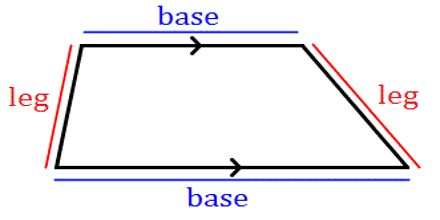Mathematic

# Lecture on TrapezoidsGeneral objective of this lecture is to present on trapezoids. A trapezoid is a quadrilateral with exactly one pair of parallel sides. The parallel sides are called bases. The nonparallel sides are called legs. At each side of a base there is a pair of base angles. The median of a trapezoid is the segment that joints the midpoints of the legs (PQ). Finally describe on Area of a trapezoid: If a trapezoid has an area of A square units, bases of b1 and b2 units and height of h units, then A = ½(b1 + b2 )h.